Created January 18, 2020 © CC BY-NC-ND

# Ada Robot Car With PID Controller

Autonomous robot with PID controller to move it between two walls.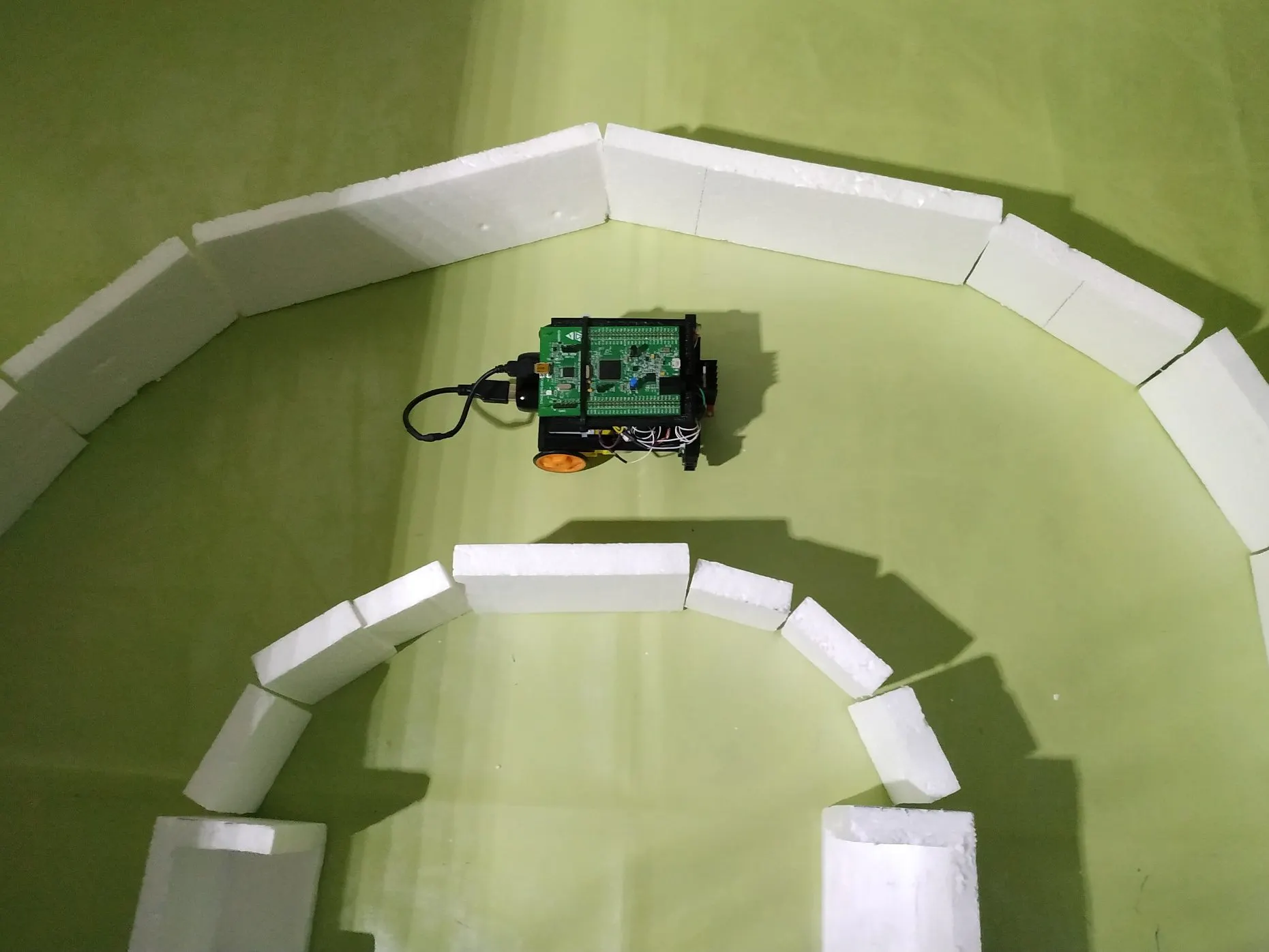## Things used in this project

### Hardware components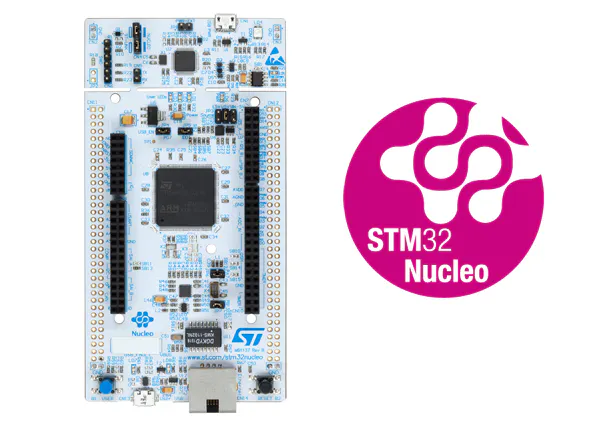ST STM32L4, STM32F7 Nucleo 144 STM32F7
×1
 SHARP IR Sensor GP2Y0A51SK0F
×2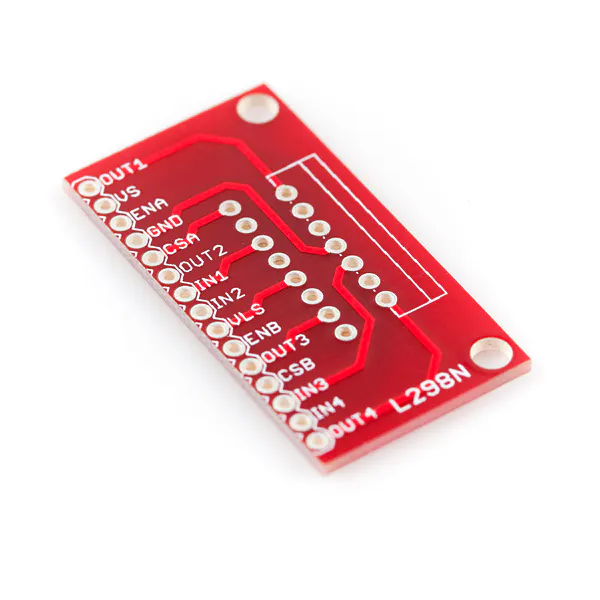SparkFun Full-Bridge Motor Driver Breakout - L298N
×1
 Gearmotor (generic)
×2
 Rechargeable battery 5V, 2A
×1
 Ball caster
×1

### Software apps and online services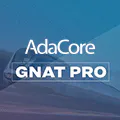### Hand tools and fabrication machines3D Printer (generic)
 Styrofoam

## Custom parts and enclosures

### STL files of: Ada Robot Car With PID Controller

In this link, you can find the same STL files of the chassis of this robot car that I designed.

## Schematics

### Schematic diagram

Electrical diagram of the autonomous robot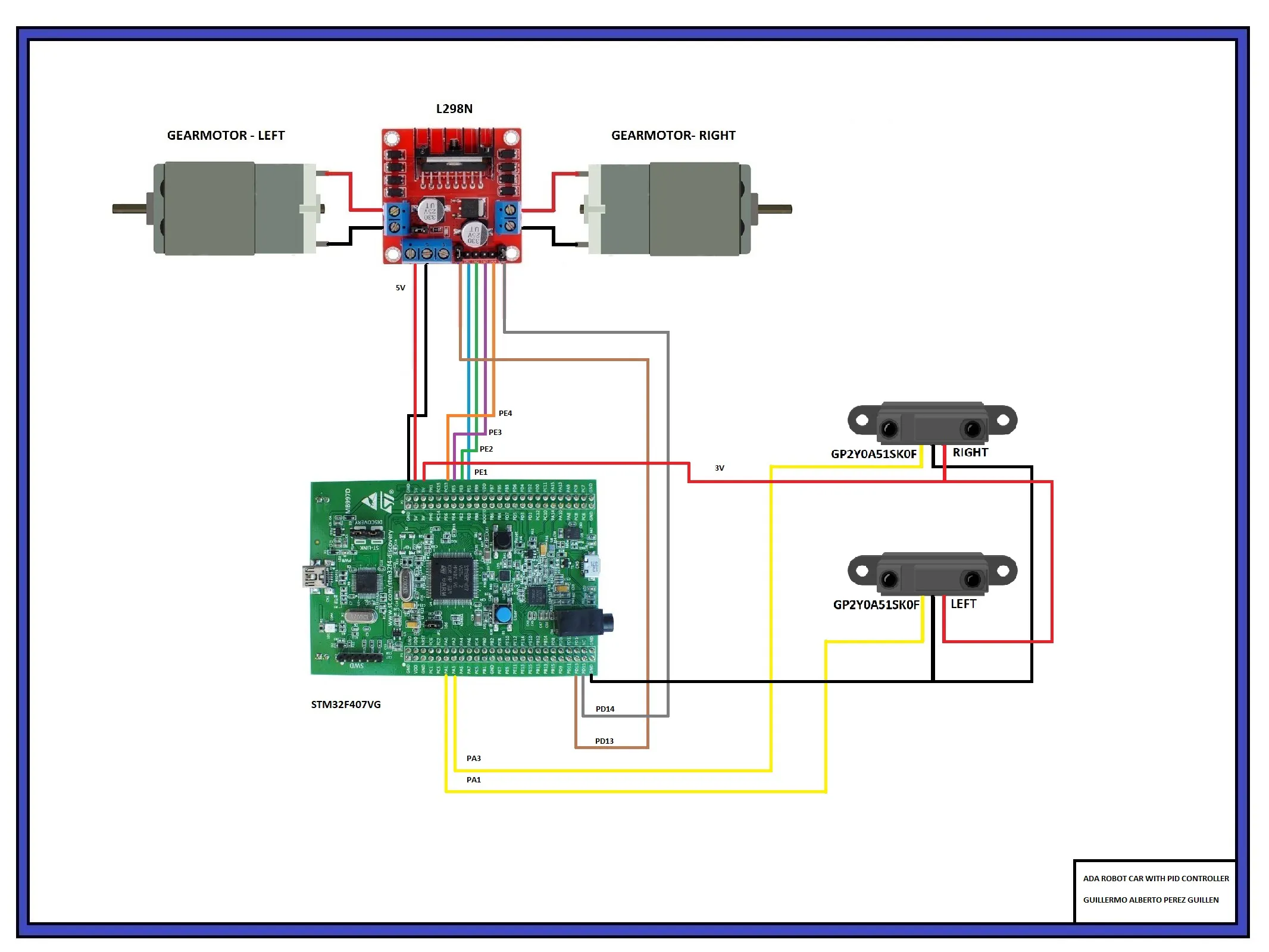## Code

This code must be compiled and uploaded to the STM32F407VG board
```-- AUTHOR: GUILLERMO PEREZ GUILLEN

with Last_Chance_Handler;  pragma Unreferenced (Last_Chance_Handler);
with STM32.Board;  use STM32.Board;
with HAL;          use HAL;
with STM32.Timers; use STM32.Timers;
with STM32.Device;  use STM32.Device;
with STM32.GPIO;    use STM32.GPIO;

----------------------------
----------------------------
Input_Channel : constant Analog_Input_Channel := 5;
Input         : constant GPIO_Point := PA1; -- analog port PA1

Input_Channel3 : constant Analog_Input_Channel := 3;
Input3        : constant GPIO_Point := PA3; -- analog port PA3

All_Regular_Conversions : constant Regular_Channel_Conversions :=
(1 => (Channel => Input_Channel, Sample_Time => Sample_144_Cycles));

All_Regular_Conversions3 : constant Regular_Channel_Conversions :=
(1 => (Channel => Input_Channel3, Sample_Time => Sample_144_Cycles));

Raw, Raw3 : UInt32 := 0;
Conv1, Conv3, Volts1, Volts3 : Long_Float;
sensor_left, sensor_right : Short_Float;

Successful : Boolean;

Period : constant := 1000;

----------------------------
-- PWM CONFIGURATIONS FOR TWO CHANNELS    --
----------------------------
Output_Channel : constant Timer_Channel := Channel_2; -- ORANGE LED -> PD13 -> Gearmotor left
Output_Channel2 : constant Timer_Channel := Channel_3; -- RED LED -> PD14 -> Gearmotor right

----------------------------
-- GPIO CONFIGURATIONS    --
----------------------------
MOTOR_1A   : GPIO_Point renames PE1; -- L298N DRIVER -> IN1
MOTOR_1B   : GPIO_Point renames PE2; -- L298N DRIVER -> IN2
MOTOR_2A   : GPIO_Point renames PE3; -- L298N DRIVER -> IN3
MOTOR_2B   : GPIO_Point renames PE4; -- L298N DRIVER -> IN4

Pattern : GPIO_Points := (PE1, PE2, PE3, PE4);

procedure Initialize_LEDs;
procedure Configure_Analog_Input;

----------------------------
-- INITIALIZE LEDs        --
----------------------------

procedure Initialize_LEDs is
begin
Enable_Clock (GPIO_E); -- GPIO LEDs

Configure_IO
(Pattern,
(Mode        => Mode_Out,
Resistors   => Floating,
Speed       => Speed_100MHz,
Output_Type => Push_Pull));

Enable_Clock (GPIO_D); -- PWM LEDs

Configure_IO
(All_LEDs,
(Mode_AF,
AF             => GPIO_AF_TIM4_2,
AF_Speed       => Speed_100MHz,
AF_Output_Type => Push_Pull,
Resistors      => Floating));

end Initialize_LEDs;

----------------------------
----------------------------

procedure Configure_Analog_Input is
begin
Enable_Clock (Input);
Configure_IO (Input, (Mode => Mode_Analog, Resistors => Floating));
end Configure_Analog_Input;

procedure Configure_Analog_Input3 is
begin
Enable_Clock (Input3);
Configure_IO (Input3, (Mode => Mode_Analog, Resistors => Floating));
end Configure_Analog_Input3;

function Sine (Input : Long_Float) return Long_Float;

----------------------------
-- FUNCTION SINE          --
----------------------------

function Sine (Input : Long_Float) return Long_Float is
Pi : constant Long_Float := 3.14159_26535_89793_23846;
X  : constant Long_Float := Long_Float'Remainder (Input, Pi * 2.0);
B  : constant Long_Float := 4.0 / Pi;
C  : constant Long_Float := (-4.0) / (Pi * Pi);
Y  : constant Long_Float := B * X + C * X * abs (X);
P  : constant Long_Float := 0.225;
begin
return P * (Y * abs (Y) - Y) + Y;
end Sine;

begin
Initialize_LEDs;

----------------------------
-- CONFIGURE TWO PWM CHANNELS --
----------------------------

Enable_Clock (Timer_4);

Reset (Timer_4);

Configure
(Timer_4,
Prescaler     => 1,
Period        => Period,
Clock_Divisor => Div1,
Counter_Mode  => Up);

Configure_Channel_Output
(Timer_4,
Channel  => Output_Channel,
Mode     => PWM1,
State    => Enable,
Pulse    => 0,
Polarity => High);

Configure_Channel_Output
(Timer_4, --
Channel  => Output_Channel2,
Mode     => PWM1,
State    => Enable,
Pulse    => 0,
Polarity => High);

Enable_Channel (Timer_4, Output_Channel);
Enable_Channel (Timer_4, Output_Channel2);

Enable (Timer_4);

----------------------------
----------------------------

Configure_Analog_Input;
Configure_Analog_Input3;

Enable_Clock (Converter);
Enable_Clock (Converter3);

Configure_Common_Properties
(Mode           => Independent,
Prescalar      => PCLK2_Div_2,
DMA_Mode       => Disabled,
Sampling_Delay => Sampling_Delay_5_Cycles);

Configure_Unit -- CONVERTER UNIT
(Converter,
Alignment  => Right_Aligned);

Configure_Unit  -- CONVERTER 3 UNIT
(Converter3,
Alignment  => Right_Aligned);

Configure_Regular_Conversions -- CONVERTER UNIT
(Converter,
Continuous  => False,
Trigger     => Software_Triggered,
Enable_EOC  => True,
Conversions => All_Regular_Conversions);

Configure_Regular_Conversions -- CONVERTER3 UNIT
(Converter3,
Continuous  => False,
Trigger     => Software_Triggered,
Enable_EOC  => True,
Conversions => All_Regular_Conversions3);

Enable (Converter);
Enable (Converter3);

declare
----------------------------
-- PID VARIABLES          --
----------------------------

Pulse, Pulse2     : UInt16;
dif : Long_Float := 0.0;
error : Long_Float := 0.0;
integral  : Long_Float := 0.0;
Kp : Long_Float := 0.01;
Kd : Long_Float := 0.01;

begin
loop
Start_Conversion (Converter);
Start_Conversion (Converter3);

Poll_For_Status (Converter, Regular_Channel_Conversion_Complete, Successful);
Poll_For_Status (Converter3, Regular_Channel_Conversion_Complete, Successful);

Raw := UInt32 (Conversion_Value (Converter)); -- LEFT SENSOR
Conv1 := Long_Float(Raw * 1);
Volts1 := (Conv1 * 0.0007326007326007326);
sensor_left := Short_Float(4.5 * (Volts1**(-1))); -- LEFT DISTANCE

Raw3 := UInt32 (Conversion_Value (Converter3)); -- RIGHT SENSOR
Conv3 := Long_Float(Raw3 * 1);
Volts3 := (Conv3 * 0.0007326007326007326);
sensor_right := Short_Float(5.0 * (Volts3**(-1))); -- RIGHT DISTANCE

dif := Conv1 - Conv3;
error := Long_Float((kp*dif)+(kd*(dif))); -- PID CONTROLLER
Pulse := UInt16 (Long_Float (Period / 2) * (1.0 - Sine (error)));
Pulse2 := UInt16 (Long_Float (Period / 2) * (1.0 + Sine (error)));
Set_Compare_Value (Timer_4, Output_Channel, Pulse); -- LEFT GEARMOTOR SPEED
Set_Compare_Value (Timer_4, Output_Channel2, Pulse2); -- RIGHT GEARMOTOR SPEED

-- GO FORWARD --
MOTOR_1A.Set;
MOTOR_1B.Clear;
MOTOR_2A.Set;
MOTOR_2B.Clear;
delay until Clock + Milliseconds (5);
end loop;
end;

```

Project data with GPS
```with "../../../../../boards/stm32f407_discovery/stm32f407_discovery_full.gpr";

for Source_Dirs use ("src");
for Object_Dir use "obj" & STM32F407_Discovery_Full.Build;
for Create_Missing_Dirs use "true";
package Builder is
end Builder;

type App_Builds is ("Debug", "Production");
App_Build : App_Builds := external ("APP_BUILD", "Production");

for Object_Dir use "obj/" & App_Build;

package Compiler is
case App_Build is
when "Debug" =>
("-g",
"-gnatwa",
"-gnata",    -- enable pre/postconditions
"-gnatQ",
"-gnatw.X",  -- disable warnings about exceptions and LCH
"-ffunction-sections",
"-fdata-sections");

when "Production" =>
"-g",
"-O2",
"-gnatp",
"-gnatn2",    -- honor Inline requests
"-Winline",  -- warn if cannot honor Inline aspect/pragma
"-gnatw.X",
"-ffunction-sections",
"-fdata-sections");
end case;
end Compiler;
```

### Projet repository: "Ada Robot Car With PID Controller"

Programming Language: AdaCore & GPS version: 19.1

## Credits

### Guillermo Perez Guillen

40 projects • 45 followers
Communications and Electronics Engineering, and Writer... "I've forgotten what I started fighting for..."